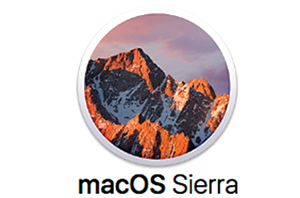S Y S T E M B E R A T U N GN E T Z W E R K EH A R D -  U N D  S O F T W A R EI N T E R N E T B E T R E U U N GP R O J E K T I O N S T E C H N I KE D V - T E C H NI S C H E  U N T E R S T Ü T Z U N GF Ü R  M E S S E N  U N D  E V E N T S I M P R E S S U M   U N D   D A T E N S C H U T Z S O  K Ö N N E N  S I E  U N S  E R R E I C H E N : T E L E F O N : 0 6 1 3 2 - 7 1 8 3 6 2 55208 Ingelheim, PSF 1519,USt-IdNr.: DE151777563